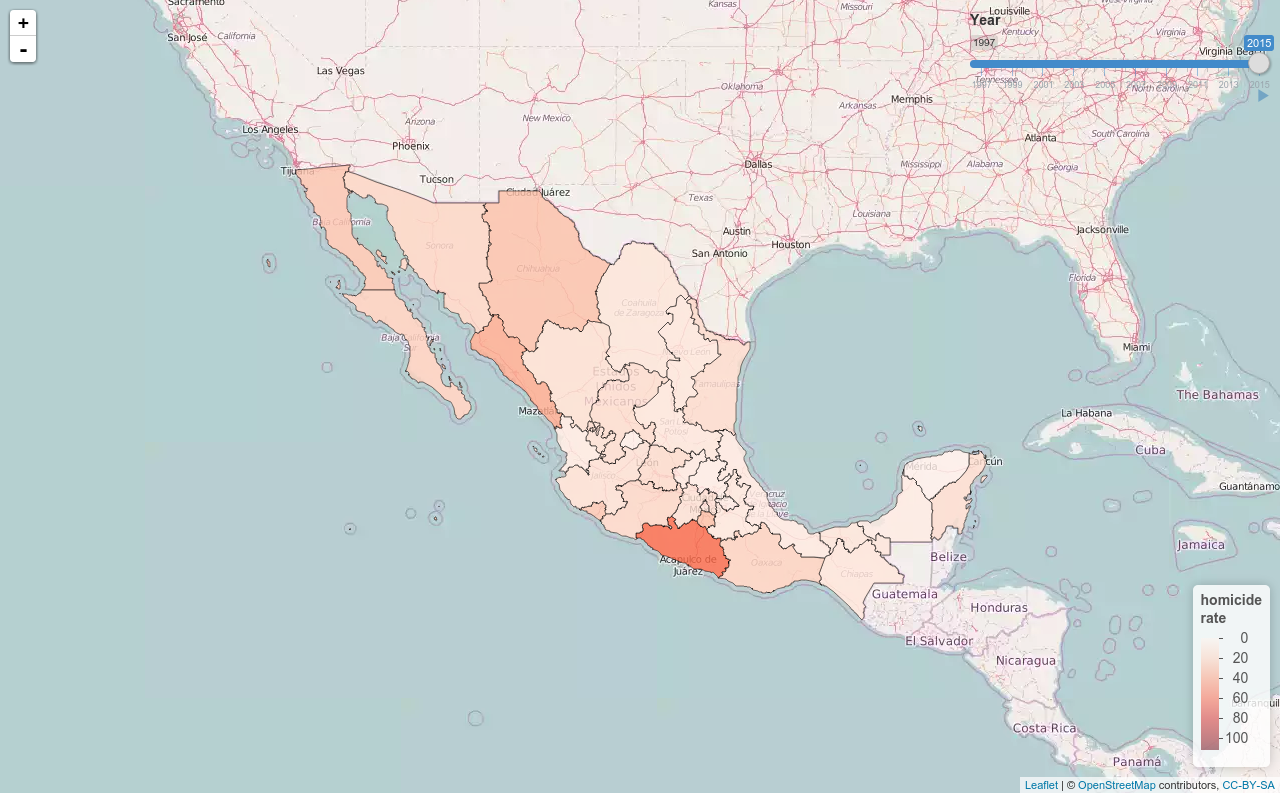You can also use the data that comes with the package to create interactive webapps with shiny.

## Load required packages
library("dplyr")
library("mxmaps")
library("geojsonio")
library("jsonlite")
library("shiny")
library("leaflet")
library("RColorBrewer")
library("lubridate")
library("zoo")
library("stringi")

## All local crimes at the state level
tmpdir <- tempdir()

## Only intentional homicides
crime <- subset(crime, modalidad == "HOMICIDIOS" & tipo == "DOLOSOS")

## subset the year from the date
crime$year <- as.integer(substr(crime$date, 1, 4))

## Yearly homicide rates
hom <- crime %>%
mutate(year = year(as.yearmon(date))) %>%
group_by(year, state_code, tipo, state) %>%
summarise(total = sum(count, na.rm = TRUE),
rate = total / mean(population) * 10^5) %>%
mutate(region = str_mxstate(state_code),
id = str_mxstate(state_code))

# Convert the topoJSON to spatial object
data(mxstate.topoJSON)
tmpdir <- tempdir()
# have to use RJSONIO or else the topojson isn't valid
write(RJSONIO::toJSON(mxstate.topoJSON), file.path(tmpdir, "state.topojson"))
# read the topojson file as an 'sf' object

# put state codes in a standard format
states$id <- str_mxstate(states$id)

ui <- bootstrapPage(
tags$style(type = "text/css", "html, body {width:100%;height:100%}"), leafletOutput("map", width = "100%", height = "100%"), absolutePanel(top = 10, right = 10, sliderInput("range", "Year", 1997, 2017, value = 2017, step = 1, sep="", animate = animationOptions(interval = 3000, loop = FALSE, playButton = NULL, pauseButton = NULL), ) ) ) server <- function(input, output, session) { # Reactive expression for the data subsetted to what the user selected filteredData <- reactive({ states <- left_join(states, subset(hom, year == input$range),
by = "id")
states
#hom[hom$year == input$range ,]
})

# This reactive expression represents the palette function,
# which changes as the user makes selections in UI.
colorpal <- reactive({
colorNumeric("Reds", hom$rate) }) output$map <- renderLeaflet({
# Use leaflet() here, and only include aspects of the map that
# won't need to change dynamically (at least, not unless the
# entire map is being torn down and recreated).
states <- left_join(states, subset(hom, year == 2017), by = "id")
pal <- colorpal()
setView(-102, 23.8, 5)%>%
pal = pal, values = ~hom\$rate,
title = "homicide<br>rate") %>%
})

# Incremental changes to the map (in this case, replacing the
# circles when a new color is chosen) should be performed in
# an observer. Each independent set of things that can change
# should be managed in its own observer.
observe({
pal <- colorpal()
leafletProxy("map", data = filteredData()) %>%
clearShapes() %>%
addPolygons(stroke = TRUE, weight = 1, color = "#000000",
fillOpacity = 0.8, smoothFactor = 0.5,
fillColor = ~pal(rate),
popup = ~ sprintf("State: %s<br/>Rate: %s",
stri_trans_totitle(state),
round(rate, 1)))
})

}

shinyApp(ui, server)